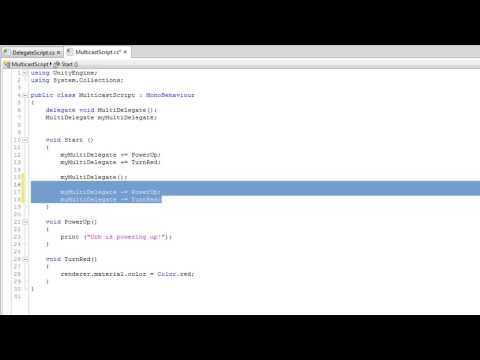Delegates

-

How to create and use delegates to provide complex and dynamic functionality in your scripts.Delegates

Intermedio Scripting

Code snippet

``````using UnityEngine;
using System.Collections;

public class DelegateScript : MonoBehaviour
{
delegate void MyDelegate(int num);
MyDelegate myDelegate;

void Start ()
{
myDelegate = PrintNum;
myDelegate(50);

myDelegate = DoubleNum;
myDelegate(50);
}

void PrintNum(int num)
{
print ("Print Num: " + num);
}

void DoubleNum(int num)
{
print ("Double Num: " + num * 2);
}
}``````
``````#pragma strict

//Javascript does not have a delegate system like
//C# does. Instead, you can create a "Function"
//container that works similarly to a delegate.
var myDelegate : Function;

function Start ()
{
myDelegate = PrintNum;
myDelegate(50);

myDelegate = DoubleNum;
myDelegate(50);
}

function PrintNum(num : int)
{
print ("Print Num: " + num);
}

function DoubleNum(num : int)
{
print ("Double Num: " + num * 2);
}``````
``````import UnityEngine
import System.Collections

public class DelegateScript(MonoBehaviour):

private callable MyDelegate(num as int) as void
private myDelegate as MyDelegate

private def Start():
myDelegate = PrintNum
myDelegate(50)

myDelegate = DoubleNum
myDelegate(50)

private def PrintNum(num as int):
print(('Print Num: ' + num))

private def DoubleNum(num as int):
print(('Double Num: ' + (num * 2)))``````

Code snippet

``````using UnityEngine;
using System.Collections;

public class MulticastScript : MonoBehaviour
{
delegate void MultiDelegate();
MultiDelegate myMultiDelegate;

void Start ()
{
myMultiDelegate += PowerUp;
myMultiDelegate += TurnRed;

if(myMultiDelegate != null)
{
myMultiDelegate();
}
}

void PowerUp()
{
print ("Orb is powering up!");
}

void TurnRed()
{
renderer.material.color = Color.red;
}
}``````
``````//Javascript's "Function" variable type does not
//allow for multicasting. To get this functionality
//you will need to use C#.``````
``````import UnityEngine
import System.Collections

public class MulticastScript(MonoBehaviour):

private callable MultiDelegate() as void
private myMultiDelegate as MultiDelegate

private def Start():
myMultiDelegate += PowerUp
myMultiDelegate += TurnRed
myMultiDelegate()

private def PowerUp():
print('Orb is powering up!')

private def TurnRed():
renderer.material.color = Color.red``````